# LaPlace's and Poisson's Equations

A useful approach to the calculation of electric potentials is to relate that potential to the charge density which gives rise to it. The electric field is related to the charge density by the divergence relationship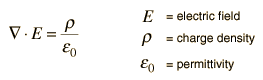and the electric field is related to the electric potential by a gradient relationship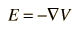Therefore the potential is related to the charge density by Poisson's equation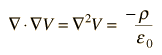In a charge-free region of space, this becomes LaPlace's equation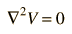This mathematical operation, the divergence of the gradient of a function, is called the LaPlacian. Expressing the LaPlacian in different coordinate systems to take advantage of the symmetry of a charge distribution helps in the solution for the electric potential V. For example, if the charge distribution has spherical symmetry, you use the LaPlacian in spherical polar coordinates.

Since the potential is a scalar function, this approach has advantages over trying to calculate the electric field directly. Once the potential has been calculated, the electric field can be computed by taking the gradient of the potential.

 Example: sphere of uniform charge
Index

Electric field concepts

 HyperPhysics***** Electricity and Magnetism R Nave
Go Back

# Potential of a Uniform Sphere of Charge

The use of Poisson's and Laplace's equations will be explored for a uniform sphere of charge. In spherical polar coordinates, Poisson's equation takes the form: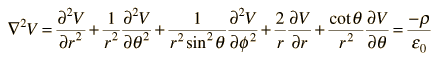but since there is full spherical symmetry here, the derivatives with respect to θ and φ must be zero, leaving the form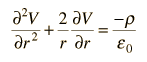Examining first the region outside the sphere, Laplace's law applies.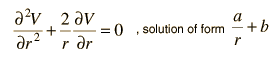Since the zero of potential is arbitrary, it is reasonable to choose the zero of potential at infinity, the standard practice with localized charges. This gives the value b=0. Since the sphere of charge will look like a point charge at large distances, we may conclude that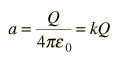so the solution to LaPlace's law outside the sphere is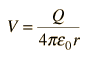Now examining the potential inside the sphere, the potential must have a term of order r2 to give a constant on the left side of the equation, so the solution is of the form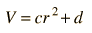Substituting into Poisson's equation gives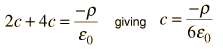Now to meet the boundary conditions at the surface of the sphere, r=R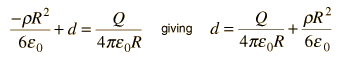The full solution for the potential inside the sphere from Poisson's equation is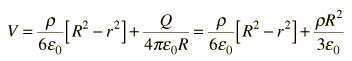Index

Electric field concepts

 HyperPhysics***** Electricity and Magnetism R Nave
Go Back